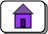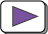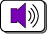# CHAPTER 90 — Introduction to Recursion

This chapter introduces recursion. Previous chapters have discussed the fundamental control structures of sequence, alternation (if-else), and iteration (while). Recursion is another control structure. Sometimes problems are stated in a recursive fashion, and a program that solves the problem is most easily written using recursion.

#### Chapter Topics:

• Two Parts to Recursion:
• If the problem is easy, solve it immediately.
• If the problem can't be solved immediately, divide it into easier problems, then solve the easier problems.
• Examples of Recursion:
• Walking a Distance
• Smashing a Rock
• Dividing a Line
• Drawing a Snowflake

Forget programming for a while. Think about a real-world problem that does not involve computers:

### QUESTION 1:

It is the day after Thanksgiving and the only parking spot you can find at the shopping mall is far from the entrance (horrors!) How do you get from your car to the mall?